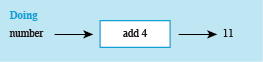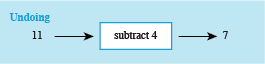Succeed with maths: part 2

Start this free course now. Just create an account and sign in. Enrol and complete the course for a free statement of participation or digital badge if available.

# 3 Doing and undoing

The next example helps you to start to think about how to work out an initial number if you know the answer and instructions using a more straightforward example than our spreadsheet. This time there are only two instructions:

• Think of a number.

If my answer is 11, can you work out what number I was thinking of? You might have said ‘What number do I have to add to 4 to get 11?’ or perhaps ‘If I take away 4 from 11, what number do I get?’. In both cases, you should have arrived at the answer 7.

In the second method, ‘subtracting 4’ undoes the ‘adding 4’ in the original instructions, and this can be illustrated with a ‘doing-undoing diagram’ (see Figure 7).Figure _unit5.3.1 Figure 7 A doing-undoing diagram

In the ‘doing’ part of the diagram, start with the number and write down the operations applied in turn until you get the answer. Here there is just one operation: ‘add 4’.

For the ‘undoing’ part of the diagram, start on the right with the answer, in this case 11. Then work back towards the left, undoing each operation in turn until you find the starting number. In this case, ‘subtract 4’ undoes ‘add 4’ and

You can then construct an undoing diagram that starts from the left, as shown in Figure 8. Notice how the arrows indicate the direction to read the diagram.Figure _unit5.3.2 Figure 8 Doing-undoing diagram

So this also shows that the number first thought of was 7.